# High School Math : Understanding Circle Functions

## Example Questions

### Example Question #3 : Center And Radius Of Circle Functions

Find the radius of the circle given by the equation: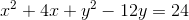Explanation:

To find the center or the radius of a circle, first put the equation in the standard form for a circle: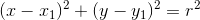, whereis the radius and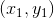is the center.

From our equation, we see that it has not yet been factored, so we must do that now. We can use the formula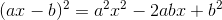.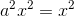, so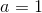.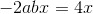and, so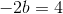and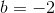.

Therefore,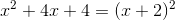.

Because the constant, in this case 4, was not in the original equation, we need to add it to both sides: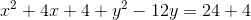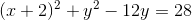Now we do the same for: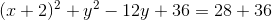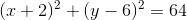We can now find: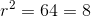### Example Question #1 : Circle Functions

Find the center of the circle given by the equation: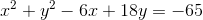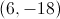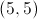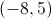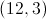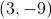Explanation:

To find the center or the radius of a circle, first put the equation in standard form:, whereis the radius andis the center.

From our equation, we see that it has not yet been factored, so we must do that now. We can use the formula., so.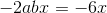and, so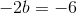and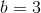.

This gives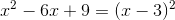.

Because the constant, in this case 9, was not in the original equation, we must add it to both sides: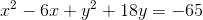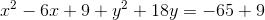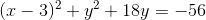Now we do the same for: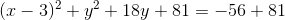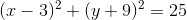We can now find the center: (3, -9)